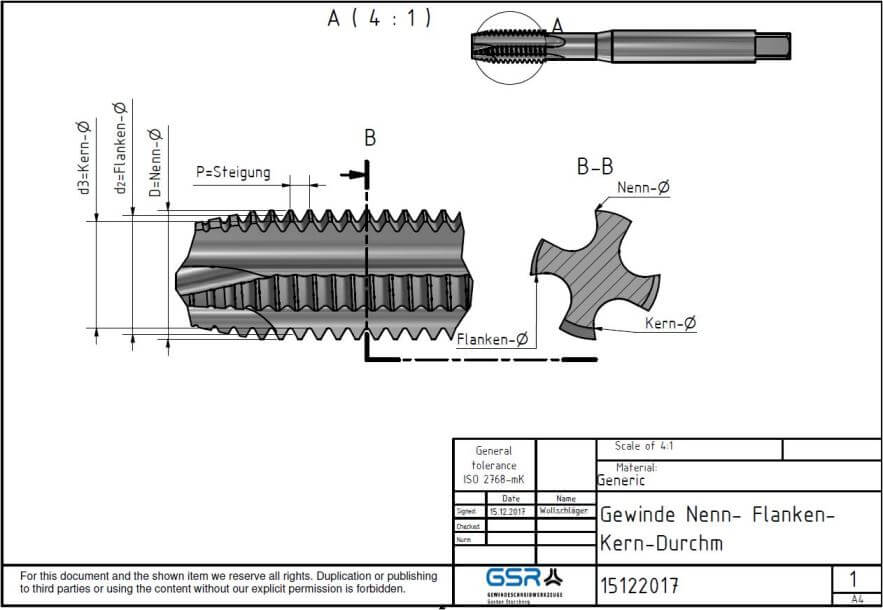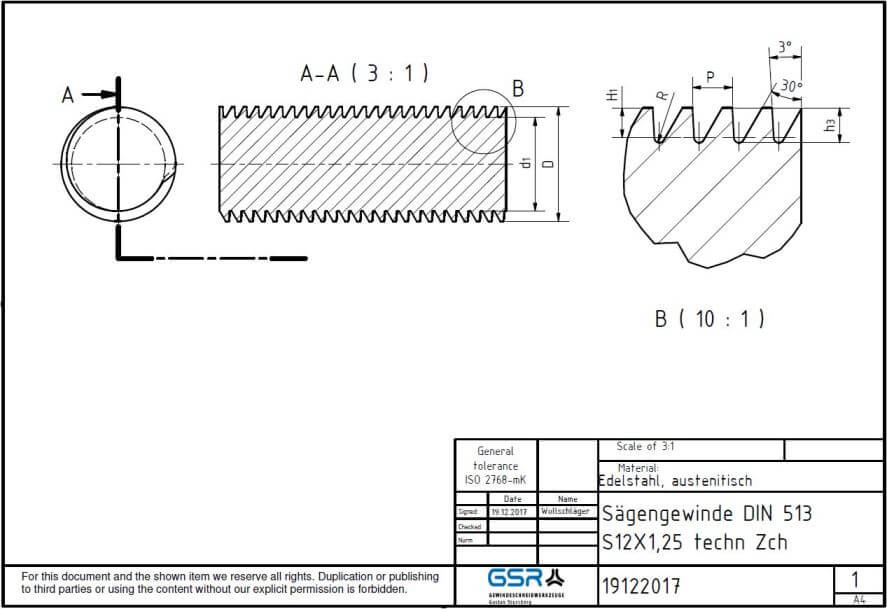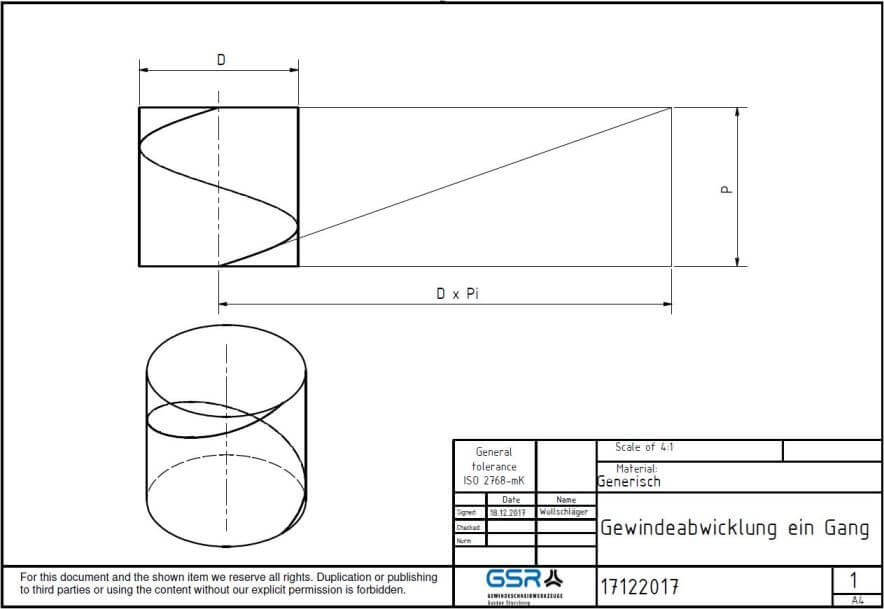In today’s article we will take a closer look at thread profiles. The main focus is on the outer diameter, the core diameter, the flank diameter.## The determinants of the diameters on a thread

A thread is essentially determined by the following diameter sizes:

### Outer diameter:

The outer diameter (d, D) is the largest diameter on a thread. The designation of a thread is determined by the outer diameter. Therefore it is also called nominal diameter. It is the diameter of an “imaginary” cylinder that encloses the profile tips of an external thread and the thread valleys of an internal thread.

### Core diameter:

The core diameter (d1, D1) is the smallest diameter on a thread. It is the diameter of an “imaginary” cylinder (inner cylinder) which excludes the profile tips of an inner thread or the profile valleys of an outer thread.

### Pitch diameter:

The flank diameter (d2, D2) is located centrally between the outer diameter and the core diameter. Among other things, it is required to determine the lead angle of a thread. It is the diameter of an “imaginary” cylinder (flank cylinder) whose cylindrical surface cuts through the thread profile in such a way that the width of the profile valleys and peaks cut out as a result is equal.

### The helix angle of a thread can be derived from the following formula:

Tan α = P / d2 x π .

The minimum and maximum dimensions of the outside diameters, the flank diameter and the core diameter are specified in the table of limit dimensions of DIN 13 – 20. The limit dimensions are listed for the common tolerance classes.## The determinants of the angles on a thread:

The profile angle β and the lead angle π determine the geometrical shape of the thread.  The lead angle of a thread can be derived from the following formula: Tan α = P / d2 x π .

The size of the flank angle determines the intended use of the thread. Fastening threads have a larger point angle, motion threads a smaller point angle. The form therefore follows the function here too. In principle, threads have three functions: they connect (screw and nut), they move (spindle in a vice) and they “strengthen” forces (jack, wine press). Let us now look at some typical thread profiles:Pointed threads have a flank angle of 60° (metric) or 55 degrees (Whitworth) and are used as fastening threads. They are designed to tighten nuts and bolts. They have a large flank angle and a small pitch angle. This results in greater friction.

Pointed threads have a flank angle of 60° (metric) or 55 degrees (Whitworth) and are used as fastening threads. They are designed to tighten nuts and bolts. They have a large flank angle and a small pitch angle. This results in greater friction.

Saw threads have a flank angle of 33° and an asymmetrical thread profile. They are therefore used where a high load is applied on one side.

A list of the most important types of threads you should know about – can be found here: 13 thread types.

##We hope you enjoyed the article.